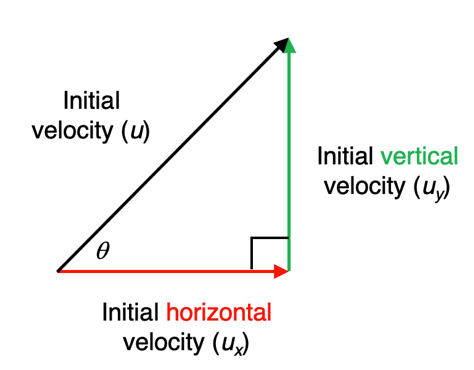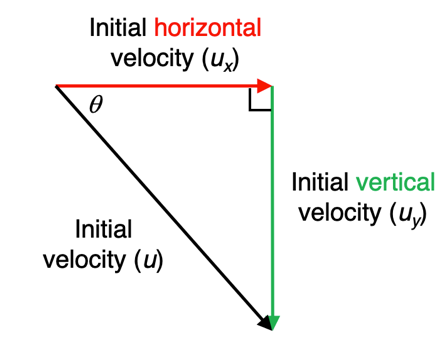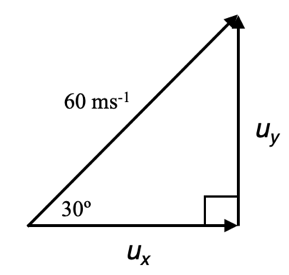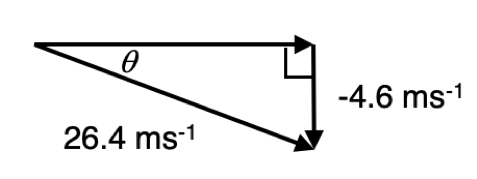# M5-S2: Full-flight Projectile Motion - Velocity and Launch Angle

• ### Apply the modelling of projectile motion to quantitatively derive the relationships between the following variables:

• initial velocity
• launch angle
• maximum height
• time of flight
• final velocity
• launch height
• horizontal range of the projectile

### Initial Velocity and Launch Angle

• All objects at the beginning of their projectile motion must possess a non-zero initial velocity.
• The initial velocity can always analysed as and resolved into two components: horizontal and vertical velocities. This is done by constructing a right-angled triangle from vectors.• Conventions for calculations
• Initial velocity is typically written as u
• Initial vertical velocity is typically written as uy – subscript y is used to represent the vertical rectilinear motion
• Initial horizontal velocity is typically written as ux – subscript x is used to represent the horizontal rectilinear motion.
• The relationship between initial velocity, initial horizontal and vertical velocity can always be represented by the right-angled triangle with q (as shown in the diagram) is the angle at which the projectile leaves the horizontal plane (usually the ground). This angle is the launch angle.

Using trigonometry, initial horizontal and initial vertical velocities can be expressed in terms of the initial velocity.

Initial horizontal velocity:

u_x/u=costheta

Initial vertical velocity:

u_y/u=sintheta

• The relationship between initial vertical and horizontal velocity is described by:

(u_y)/(u_x)=tantheta

• Since the triangle is right-angled, the three vectors’ relationship can also be summarised by Pythagoras’s theorem.

u^2=(u_x)^2+(u_y)^2

u=sqrt((u_x)^2+(u_y)^2)

The initial velocity can be negative because the initial direction of a projectile can also be downwards as shown below. Situations in which this type of initial velocity occurs will be explored and clarified in practice questions later.## Practice Question 1

A projectile is launched at 60 ms-1 at an elevation of 300. Find its initial horizontal and vertical velocities.

Construct a right-angled triangle from vectors:Initial horizontal velocity:

u_x=60cos30^o

Initial vertical velocity:

u_y=60sin30^o

• Initial vertical velocity changes throughout projectile motion. Its magnitude decreases when a object travels upwards and increases when it travels downwards.
• Initial horizontal velocity remains constant and does not change.

### Changes in Vertical Velocity

• Motion in the vertical axis can be modelled using rectilinear equations. In contrast, motion in the horizontal axis does not require these equations because horizontal acceleration is zero.
s=u_yt+1/2a_yt^2

v_y=u_y+a_yt

v_y^2=u_y^2+2a_ys

s represents the vertical displacement, ay represents the acceleration in the vertical axis (gravity), uy and vy are the initial vertical velocity and velocity after time t of the object during its projectile motion.

## Practice Question 2

The initial vertical velocity of an object during projectile motion is 15 ms-1. The launch angle is 30^o.

(a) Calculate the initial horizontal velocity.

u_y/u_x=tantheta

u_x=u_y/tantheta

u_x=15/tan30^o

u_x=25.98  ms^-1

(b) Calculate the vertical velocity and the instantaneous velocity 2 seconds after the object’s launch.

The vertical velocity can be modelled by the following equation:

v_y=u_y+a_yt

Since, acceleration due to gravity is acting against the direction of the object, a negative sign must be placed in front of 9.8.

v_y=15+(-9.8)(2)

v_y=-4.6  ms^-1

The instantaneous velocity can be determined using Pythagoras’s theorem:

v=sqrt(v_x^2+v_y^2)

v=sqrt((25.98)^2+(-4.6)^2)

v=26.4  ms^-1

Since velocity is a vector quantity, a direction is needed. The direction is typically indicated by the angle relative to the horizontal.Using trigonometry:

v_y/v=sintheta

4.6/26.4=sintheta

theta=sin^-1(4.6/26.4)

theta=10.0^o

Therefore, the instantaneous velocity of the object 2 seconds after launch is 26.4 ms-1 10.0° relative to the horizontal axis.

• Construction of a right-angled triangle using velocity vectors can be done at any point during an object’s projectile motion.
• The horizontal velocity (horizontal vector) remains constant and does not change in length.
• The angle, vertical velocity (vertical vector) and the instantaneous velocity (hypotenuse) change with time.

Previous section: Introduction to Projectile Motion

Next section: Full-flight Projectile Motion - Maximum Height, Time of Flight and Range